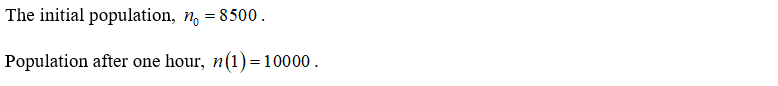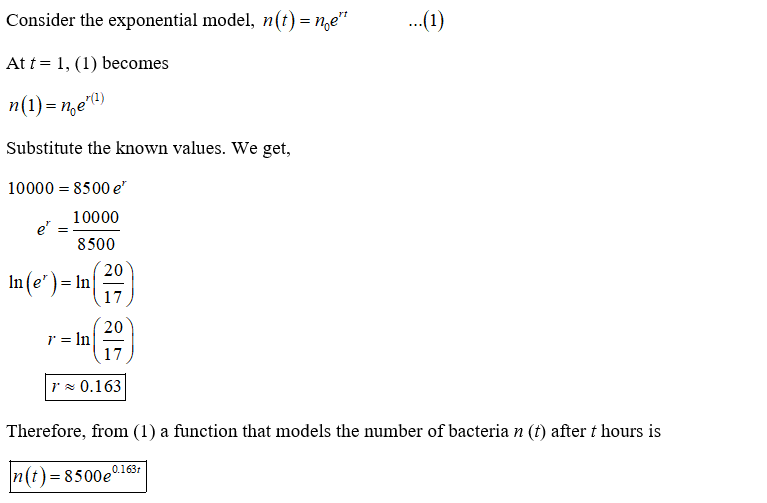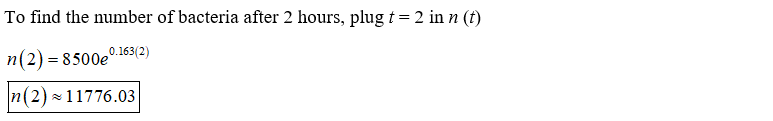# This exercise uses the population growth model.A culture starts with 8500 bacteria. After 1 hour the count is 10,000.(a) Find a function that models the number of bacteria n(t) after t hours. (Round your r value to three decimal places.)n(t) =  (b) Find the number of bacteria after 2 hours. (Round your answer to the nearest hundred.)(c) After how many hours will the number of bacteria double? (Round your answer to one decimal place.)

Question
34 views

This exercise uses the population growth model.

A culture starts with 8500 bacteria. After 1 hour the count is 10,000.

(a) Find a function that models the number of bacteria n(t) after t hours. (Round your r value to three decimal places.)
n(t) =

(b) Find the number of bacteria after 2 hours. (Round your answer to the nearest hundred.)

(c) After how many hours will the number of bacteria double? (Round your answer to one decimal place.)

check_circle

Given:Part a)Part b)...

### Want to see the full answer?

See Solution

#### Want to see this answer and more?

Solutions are written by subject experts who are available 24/7. Questions are typically answered within 1 hour.*

See Solution
*Response times may vary by subject and question.
Tagged in